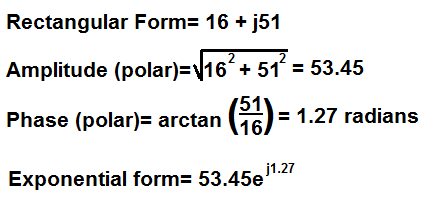﻿ Rectangular to Exponential Form Conversion Calculator ﻿# Rectangular to Exponential Form Conversion Calculator

FormulaExampleEnter the Real Number Enter the Imaginary Number j

This rectangular to exponential form conversion calculator converts a number in rectangular form to its equivalent value in exponential form.

Rectangular forms of numbers take on the format, rectangular number= x + jy, where x and y are numbers. The x is the real number of the expression and the y represents the imaginary number of the expression.

Exponential forms of numbers take on the format, re, where r is the amplitude of the expression and θ is the phase of the expression. The amplitude r must be expressed in absolute value form. R cannot be a negative number. θ (theta) must be expressed in unit radians. Unlike the polar form, which is expressed in unit degrees, a complex exponential number is expressed in unit radians.

Rectangular forms of numbers can be converted into their exponential form equivalents by the formula, Polar amplitude= √ x2 + y2 , where x and y represent the real and imaginary numbers of the expression in rectangular form. This finds the amplitude of the polar expression.

To find the phase of the exponential form, the formula to do so is, phase= arctan(y/x), where y is the imaginary number and x is the real number. This calculator computes the phase in radians, not degrees, because in exponential form, the phase is always expressed in unit radians. This is opposed to polar form, in which the phase is expressed in unit degrees.

So, for example, let's take the expression in rectangular form, 12 + 87j. We want to convert this into its equivalent exponential form. So, to do this, we take the formula and plug in the values, giving us for the amplitude, amplitude = √ 122 + 872 = 87.82. For the phase, we plug the numbers into the formula, giving us for the phase, phase= arctan(87/12)= 1.43.

And that's all that's required in order to convert from rectangular form to exponential form.

Since the only 2 components of a number expressed in rectangular form are the real number and the imaginary number, these are the only 2 inputs required for the calculator to compute the equivalent number in exponential form.

Related Resources

﻿